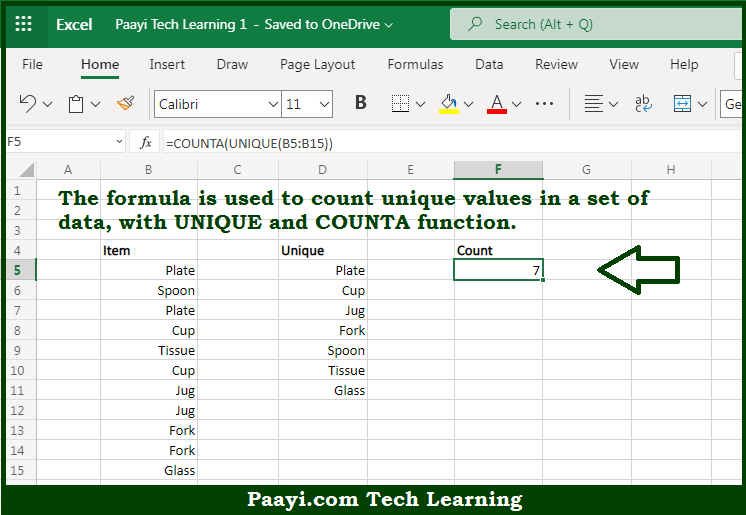# Learn How to Count Unique Values in Microsoft Excel

Written by | 0 Comments | 658 Views

In this article, you will learn how to evaluate things in Dynamic Arrays with formulas in Microsoft Excel using a single/combination(s) of functions. You will also know How to Count Unique Values and see the generic formula.

How to Count Unique Values in Microsoft Excel

The main purpose of this formula is to count unique values in a set of data. Here we will learn how to count unique values from the given data set in the workbook in Microsoft Excel. That implies, with the help of a formula based on the UNIQUE and COUNTA function you can able to count unique values in a set of data. So, with the help of this formula, you can able to count unique values from the given data set in the workbook in Microsoft Excel.

General Formula to Count Unique Values

=COUNTA(UNIQUE(data))

The Explanation for the Count Unique ValuesSo we know that with the help of the given formula above you can able to count unique values in a set of data. Here we will learn how to count unique values from the given data set in the workbook in Microsoft Excel. As we know that the COUNT function, is used to count only numbers, while the COUNTA function counts both text and numbers. In the example provided, there are seven items in the array. This formula is dynamic and will recalculate immediately when source data is changed. So, with the help of this formula, you can able to count unique values in a set of data. Here we will learn how to count unique values from the given data set in the workbook in Microsoft Excel.